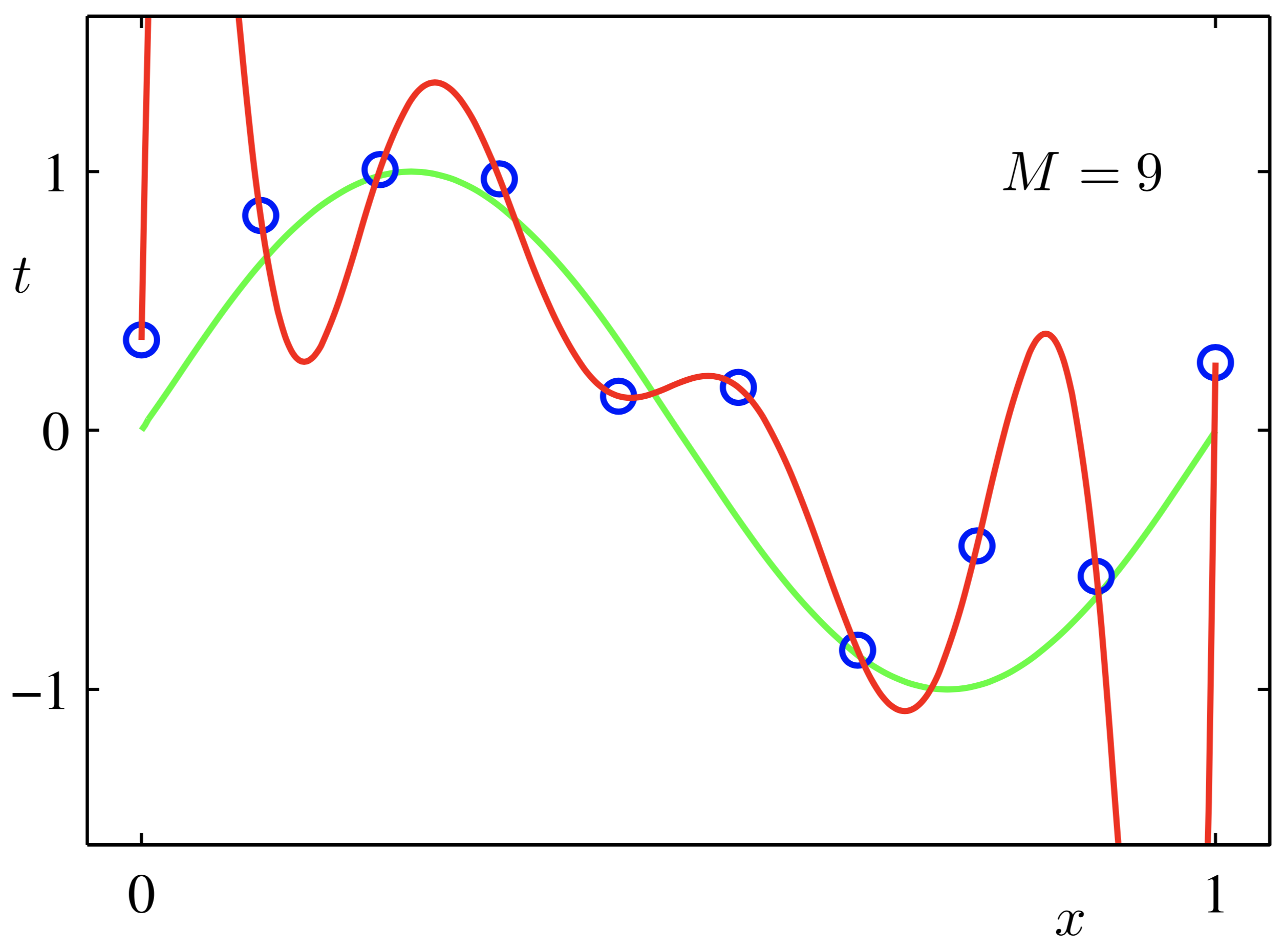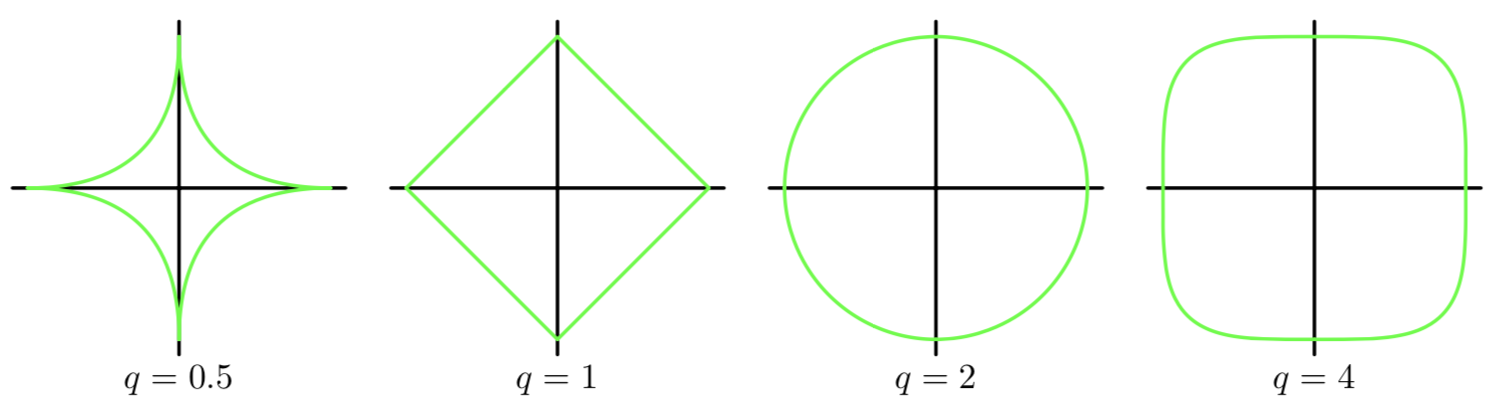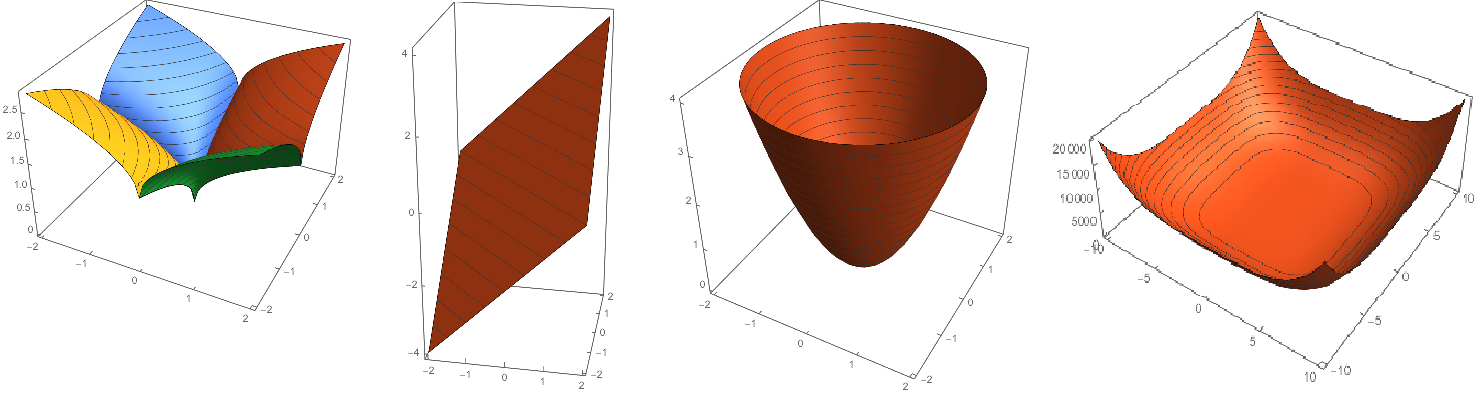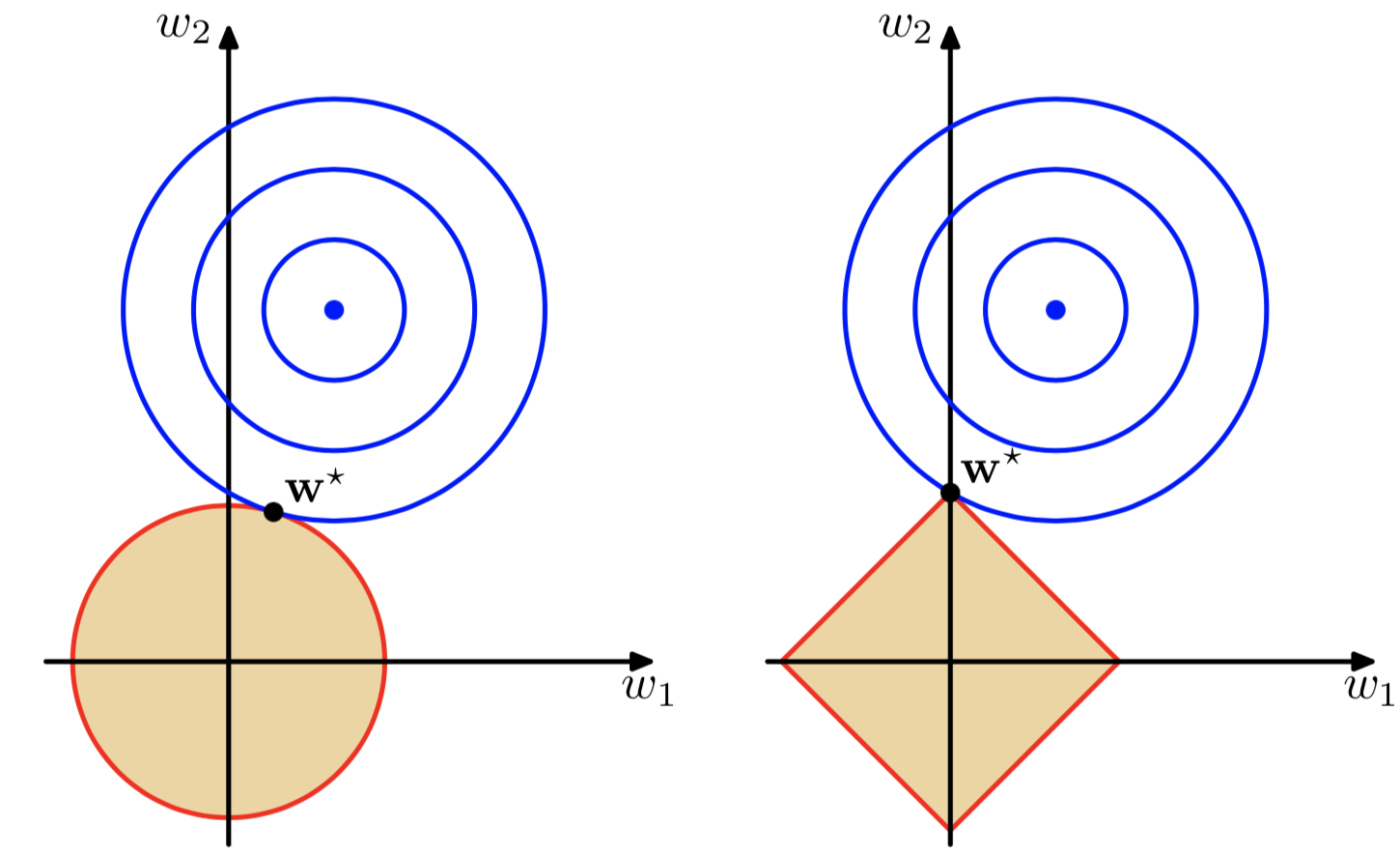【阅读时间】7min - 9min
【内容简介】主要解决什么是正则化，为什么使用正则化，如何实现正则化，外加一些对范数的直观理解并进行知识整理以供查阅

# Why & What 正则化# How 线性模型角度

## 二次正则项

$$E(\mathbf w) =\frac{1}{2} \sum_{n=1}^{N}\{t_n-\mathbf w^T \phi (\mathbf x_n)\}^2 \tag{1}$$

$E(\mathbf w)$ 是损失函数（又称误差函数）E即Evaluate，有时候写成L即Loss
$t_n$ 是测试集的真实输出，又称目标变量【对应第一幅图中的蓝色点】
$\mathbf w$ 是权重（需要训练的部分，未知数）
$\phi()$ 是基函数，例如多项式函数，核函数

$$\frac{1}{2} \sum_{n=1}^{N}\{t_n-\mathbf w^T \phi (\mathbf x_n)\}^2 + \frac{\lambda}{2} \mathbf w^T \mathbf w \tag{2}$$

(2)式被称为目标函数（评价函数）= 误差函数（损失函数） + 正则化项
$\lambda$ 被称为正则化系数，越大，这个限制越强

2式对 $\mathbf w$ 求导，并令为0（使误差最小），可以解得

$$\mathbf w = (\lambda \mathbf I + \Phi^T \Phi)^{-1}\Phi^T\mathbf t$$

## 一般正则项

$$\frac{1}{2} \sum_{n=1}^{N}\{t_n-\mathbf w^T \phi (\mathbf x_n)\}^2 + \frac{\lambda}{2} \sum_{j=1}^{M} {\vert w_j \vert}^q \tag{3}$$

M是模型的阶次（表现形式是数据的维度），比如M=2，就是一个平面（二维）内的点

q=2就是二次正则项。高维度没有图像表征非常难以理解，那就使用二维作为特例来理解。这里令M=2，即 $\mathbf x =\{x_1,x_2\} \;\mathbf w=\{w_1,w_2\}$ ，令q=0.5 q=1 q=2 q=4q=2是一个圆非常好理解，考虑 $z = w_1^2 + w_2^2$ 就是抛物面，俯视图是一个圆。其他几项同理（必须强调俯视图和等高线的概念，z轴表示的是正则项项的值）$w^*$ 最小值取到的点

# How 神经网络模型角度

M表示单层神经网中隐含层中的神经元的数量

# 范数

## 向量范数### p-范数

$$\Vert\mathbf x \Vert_p =(\sum\limits_{i=1}^{N}\vert x_i \vert^p)^{\frac{1}{p}}$$

### $-\infty$-范数

$$\Vert \mathbf x \Vert_{-\infty} = arg \operatorname*{min}_{i}{\vert x_i \vert}$$

### 1-范数

$$\Vert \mathbf x \Vert_1 = \sum\limits_{i=1}^{N}\vert x_i \vert$$

$$\begin{matrix} 4 & 3 & 2 & 3 & 4 \\ 3 & 2 & 1 & 2 & 3\\ 2 & 1 & 0 & 1 & 2 \\ 3 & 2 & 1 & 2 &3 \\ 4&3 & 2 &3 &4 \\ \end{matrix}$$

### 2-范数

$\Vert\mathbf x \Vert_2 = \sqrt{\sum\limits_{i=1}^{N} x_i^2}$ ：向量元素的平方和再开方Euclid范数，也称欧几里得范数，欧氏距离

$$\begin{matrix} 2.8&2.2&2&2.2&2.8 \\ 2.2&1.4&1&1.4&2.2 \\ 2&1&0&1&2 \\ 2.2&1.4&1&1.4&2.2 \\ 2.8&2.2&2&2.2&2.8 \\ \end{matrix}$$

### $\infty$-范数

$\Vert \mathbf x \Vert_{\infty} = arg \operatorname*{max}_{i}{\vert x_i \vert}$ ：所有向量元素中绝对值的最大值，也称棋盘距离（chessboard），切比雪夫距离

$$\begin{matrix} 2 & 3 & 2 & 2 & 2 \\ 2 & 1 & 1 & 1 & 2\\ 2 & 1 & 0 & 1 & 2 \\ 2 & 1 & 1 & 1 &2 \\ 2&2 & 2 &2 &2 \\ \end{matrix}$$

## 矩阵范数

### 1-范数

$$\Vert \mathbf A \Vert_{1} = arg \operatorname*{max}_{1 \leqslant j \leqslant n}\sum\limits_{i=1}^m{\vert a_{i,j} \vert}$$

### $\infty$-范数

$$\Vert \mathbf A \Vert_{\infty} = arg \operatorname*{max}_{1 \leqslant i \leqslant n}\sum\limits_{j=1}^m{\vert a_{i,j} \vert}$$

### 2-范数

$\Vert \mathbf A \Vert_{2} = \sqrt{\lambda_{max}(\mathbf A^* \mathbf A) }$

p=2m=n方阵时，称为谱范数。矩阵 $\mathbf A$ 的谱范数是 $\mathbf A$ 最大的奇异值或半正定矩阵 $\mathbf A^T \mathbf A$ 的最大特征值的平方根

$\mathbf A^*$ 为 $\mathbf A$ 的共轭转置，实数域等同于 $\mathbf A^T$

### F-范数

$\Vert \mathbf A \Vert_{F} = \sqrt{ \sum\limits_{i=1}^m \sum\limits_{j=1}^n \vert a_{i,j}\vert^2 }$

Frobenius范数（希尔伯特-施密特范数，这个称呼只在希尔伯特空间），即矩阵元素绝对值的平方和再开平方

### 核范数

$\Vert \mathbf A \Vert_{*} = \sum\limits_{i=1}^n \lambda_i$ ：$\lambda_i$ 若 $\mathbf A$ 矩阵是方阵，称为本征值。若不是方阵，称为奇异值，即奇异值/本征值之和

# 总结

Learning with intuitive and get Insight